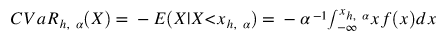# Python风险价值计算投资组合VaR（Value at Risk ）、期望损失ES（Expected Shortfall）

Python计算获得多资产投资组合的风险度量。

# 关键概念

1. 风险本身被看作是实际收益和期望收益之间的差异，两者可能不同。如果它们相等，投资被认为是无风险的。同时，它不能有违约风险，也不能有再投资风险。请注意，期望收益不是投资者认为他们将获得的收益，而是反映了所有经济情况下所有可能结果的平均值。
2. 风险价值（VaR）告诉你在一个给定的时间段内，在预先确定的置信水平下，你能损失多少钱。典型的置信度是95%和99%，意味着分析师有95%或99%的信心，损失不会超过这个数字，即5%（或1%）的VaR反映了5%（或1%）最坏情况下的未来最佳收益率。风险值是一个最先进的衡量标准，因为它可以为所有类型的资产进行计算，并考虑到多样化的因素。然而，风险值并不是一个最大的损失数字，所以分析师可能会遇到大于风险值的损失。
3. 关于历史序列的假设
• 过去的收益率是未来收益率的预测指标，但不能保证历史记录会显示未来最坏和最好的情况，但我们用几何平均法将价格转化为收益，所以我们对所有不同的周/月/…收益给予同等的权重，来获得T年内投资收益的复合最终价值。
• 如果资产价格中的期望收益是合理的，那么实际收益率应该围绕这些预期呈正态分布。当收益率可以很好地接近于正态分布时，投资管理就变得更加容易操作了。

# R语言风险价值VaR（Value at Risk）和损失期望值ES（Expected shortfall）的估计

``````#VaR计算在Python中的应用

#准备工作（每个库都要用 "pip install \*libraryname\*"来预安装
import pandas as pd
import numpy as np
import matplotlib.pyplot as plt

#从雅虎财经下载谷歌数据到定义的时间段内

#收益率的计算
df\['return'\] = Close.pct_change()

#VaR计算
VaR\_90 = norm.ppf(1-0.9, mean, std\_dev)

print('VaR 90%置信度: ', VaR_90)``````

### 随时关注您喜欢的主题

``````#准备工作
import numpy as np
import pandas as pd
#从Quandl API导入银行数据（.4表示收盘价）。
ticker = "WIKI/BAC.4"
quandl.get(ticker,

#以升序方式呈现数据
sorted( percentage\["Close"\])

print ("99.99%的实际损失不会超过" ,percentile(order_percentage, .01) * 100)``````

# 多资产投资组合VaR

``````#将数据集扩展到5种不同的资产，将它们组合成一个具有替代风险的投资组合。
\[ "WIKI/NKE.4", "WIKI/NFLX.4", "WIKI/AMZN.4"\]

#收益率的计算
df.pct_change()

#不同的风险敞口进入投资组合
percentage * exposures
ptf\_percentage = value\_ptf\['投资组合的价值'\] 。
np.percentile(ptf_percentage, .01)

print ("99.99%的实际损失不会超过：" round(VaR, 2)

print ("预计损失将超过" + (ptf\_percentage)) + "超过" ptf\_percentage)) + "天数")``````

# 在搜索VaR相关文献时，你会发现有很多关于VaR作为市场风险衡量标准的批评意见。你不可避免地看到期望损失（ES）被提出来作为一种替代。

VaR回答了问题:在1%的尾部，整个结果范围内的最小损失是多少？
ES回答了问题:在1%的尾部，整个结果范围内的平均损失是多少？

# VaR

``````h = 10. # 为10天
mu_h = 0.1 # 这是10天内收益率的平均值 - 10%。
sig = 0.3 # 这是一年内收益率的波动 - 30%。

VaR\_n = normppf(1-alpha)*sig\_h - mu_h``````

# 条件VaR/期望损失EXPECTED SHORTFALL``````# 与上述参数相同
alpha**-1 * norm.pdf(norm.ppf(alpha))*sig\_h - mu\_h``````

``````nu = 5 # 自由度，越大，越接近于正态分布
print("99% CVaR ", (CVaR_t*100,2)``````

``````# 验证正态分布和Student-t VaR是一样的
nu = 10000000 # 自由度，越大，越接近于正态分布

print("99% VaR", round(VaR_t*100,2))``````

``mu\_norm, sig\_norm = norm.fit(returns``

## 绘制具有不同自由度的VaR和CVaR图表

``````plt.plot(d\[0\], d\[1\]*100
plt.plot(np.arange(5, 100), VaR_n\*np.ones(95)\*100``````
``VaR\_n = norm.ppf(1-alpha)*sig\_norm -munorm``

Python确实是一个强大的工具，用于计算和数据可视化。它允许你导入几个不同的预包装库，大大降低了其他代码（如C++）的复杂性。

Kaizong Ye拓端研究室（TRL）的研究员。

​非常感谢您阅读本文，如需帮助请联系我们！QQ在线咨询

15121130882

0571-63341498

## 关注有关新文章的微信公众号

This will close in 0 seconds